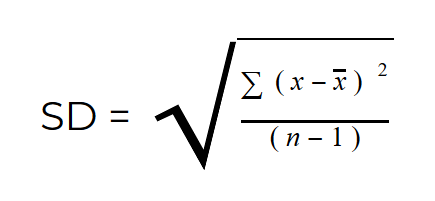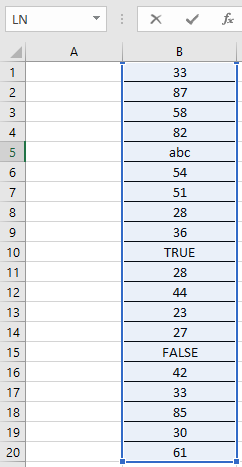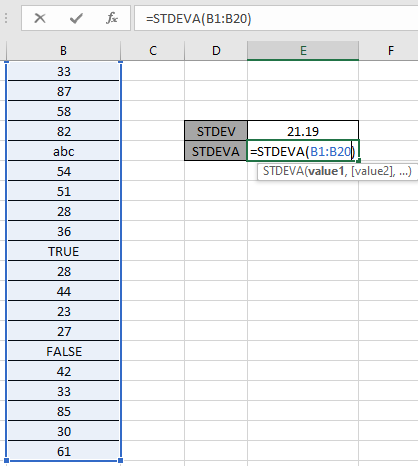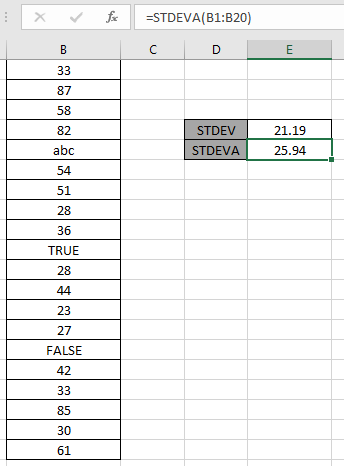# How to use the STDEVA Function in Excel

In this article, we will learn about how to use STDEVA function in Excel to calculate the standard deviation for the range of values.

STDEVA function is a built-in function to calculate the standard deviation of the range of data provided. The standard deviation for the set of values is calculated using the formula shown below.Here

1. x is the mean or average of the data.
2. n is the number of values in the data.

There are newer and updated versions of this function used for a different set of values.

1. STDEVP: This function used for data set having population
2. STDEV.S: This function used for data set have sample values
3. STDEV.P: This function is newer version for STDEVP. Excel recommends this over STDEVP
4. STDEVA: This function accepts the text or cell reference values which other STDEV functions ignore.
5. STDEVPA: This function accepts population as data set and text and logic_values.

Logica values : TRUE is considered as 1 whereas FALSE as 0.

Text : text values are considered as 0.

STDEVA function in excel returns a number which is the standard deviation, considering data having text or logical values.

Syntax of STDEVA function:

 =STDEVA ( Value 1, [value 2], ...)

value 1 : First value ( necessary )

value 2 : Second value ( optional ). Upto 255 values can be added.

Example:

Let’s understand this function using it in an example.Here we have a range of values from B1 : B20 cell and we need to get the standard deviation for the same using the below formula

Use the formula

 =STDEVA ( B1 : B20 )Here array argument to the function is given using the cell reference method.

Using the above formula in the cell to get the standard deviation of the values.The Standard deviation for the range considering text values and Logic values is 25.94 whereas STDEV function returns 21.19 . This difference occurs as the value of n in the formula changes, as in STDEVA case value of n is 20 ( includes text & logic values )  but for STDEV case value of n is 17 ( ignores text & Logic values ).

Notes:

1. The function is used for the sample data.
2. If data represents a Population, use the STDEVPA function. STDEVPA function is used to calculate the standard deviation for an entire population considering text and logical values.
3. Arguments which are error values, returns error.
4. The function returns the #DIV/0! (division) error, if there’s only text or logic values.

As you can see the STDEVA function in excel returns values from the table field.

Hope you understood How to use the STDEVA function in Excel. Explore more articles on Mathematical functions like STDEV and STDEV.P functions here. Please state your query or feedback in the comment box below.

Related Articles

How to use the STDEV.P function in Excel

How to use the STDEV function in Excel

How to use STDEV.S function in Excel

Popular Articles

Edit a dropdown list

If with conditional formatting

Vlookup by date

Terms and Conditions of use

The applications/code on this site are distributed as is and without warranties or liability. In no event shall the owner of the copyrights, or the authors of the applications/code be liable for any loss of profit, any problems or any damage resulting from the use or evaluation of the applications/code.